Given a line and a point, construct a line through the point, parallel to the given line.

 1. Begin with point P and line k.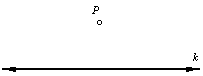2. Draw an arbitrary line through point P, intersecting line k. Call the intersection point Q. Now the task is to construct an angle with vertex P, congruent to the angle of intersection.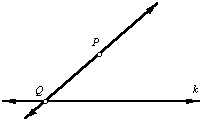3. Center the compass at point Q and draw an arc intersecting both lines. Without changing the radius of the compass, center it at point P and draw another arc.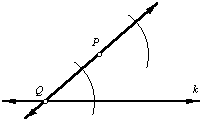4. Set the compass radius to the distance between the two intersection points of the first arc. Now center the compass at the point where the second arc intersects line PQ. Mark the arc intersection point R.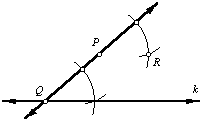5. Line PR is parallel to line k.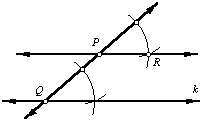Back to Geometry Construction Reference